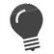# FP_CMP

Compare floating-point numbers

 WTSupported in traditional Synergy on Windows USupported on UNIX VSupported on OpenVMS
```xcall FP_CMP(result, float1, float2)
```

Arguments

result

Returned with one of the following values: (n)

0 = Float1 equals float2.

-1 = Float1 is less than float2.

1 = Float1 is greater than float2.

float1

A native floating-point number to compare to float2. (a8)

float2

A native floating-point number to be compared to float1. (a8)

Discussion

The FP_CMP subroutine compares two floating-point numbers to see if they are equal or if one is greater than the other.

Result, float1, and float2 are native floating-point numbers represented as a8 variables.

This routine can also be called as a function.Before you can use a subroutine as a function, you must first declare it external:```external function fp_cmp ,d ``` Then you can use FP_CMP as follows:```if (%fp_cmp(float1, float2)) then writes(TTCHN, "not equal") else writes(TTCHN, "equal") ```